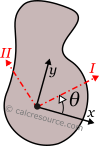## Principal Moments of Inertia

This tool calculates the principal moments of inertia (second moment of area) of a planar shape, given its moments of inertia to known axes. Enter the moments of inertia Ixx, Iyy and the product of inertia Ixy below. The calculated results will have the same units as your input. Please use consistent units for any input.

 Ixx = Iyy = Ixy = The Principal Moments of Inertia are: II = III = θ =ADVERTISEMENT

## Definitions

### Principal axes

In principal axes, that are rotated by an angle θ relative to original centroidal ones x,y, the product of inertia becomes zero. Because of this, any symmetry axis of the shape, is also a principal axis. The moments of inertia about principal axes, are called principal moments of inertia, and are the maximum and minimum ones, for any angle of rotation of the coordinate system. If Ix, Iy and Ixy are known for the arbitrary centroidal coordinate system x,y, then the principal moments of inertia and the rotation angle θ of the principal axes can be found, through the next expressions:

### Dimensions

The dimensions of moment of inertia (second moment of area) are .

### Mass moment of inertia

In Physics the term moment of inertia has a different meaning. It is related with the mass distribution of an object (or multiple objects) about an axis. This is different from the definition usually given in Engineering disciplines (also in this page) as a property of the area of a shape, commonly a cross-section, about the axis. The term second moment of area seems more accurate in this regard.

### Applications

The moment of inertia (second moment or area) is used in beam theory to describe the rigidity of a beam against flexure (see beam bending theory). The bending moment M applied to a cross-section is related with its moment of inertia with the following equation:

where E is the Young's modulus, a property of the material, and κ the curvature of the beam due to the applied load. Beam curvature κ describes the extent of flexure in the beam and can be expressed in terms of beam deflection w(x) along longitudinal beam axis x, as: . Therefore, it can be seen from the former equation, that when a certain bending moment M is applied to a beam cross-section, the developed curvature is reversely proportional to the moment of inertia I. Integrating curvatures over beam length, the deflection, at some point along x-axis, should also be reversely proportional to I.